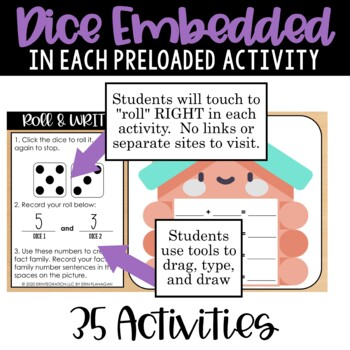1st
Subjects
Standards
Resource Type
Formats Included
• PDF
•Google Apps™The Teacher-Author indicated this resource includes assets from Google Workspace (e.g. docs, slides, etc.).

### Description

Easy to assign digital dice games and activities to practice first grade math skills. 35 activities are preloaded right onto Seesaw™ to save you time. A Google™ Slides version is also included to assign on Google Classroom.

Features real working dice RIGHT on the activity slide. NO links for students to click or additional sites that students need to visit! Students just touch the dice to "roll" and touch again to stop. The dice resets automatically when students click elsewhere on the canvas.

This version features games designed with 4 digital dice types: single traditional 6 sided dice, double dice, 3D shapes dice, and time to the nearest half hour dice.

Activities are designed to be completed independently, which makes them great for distance learning, hybrid learning, homework, or as a math center in the classroom.

Interactive Games Included:

• Roll & Cover
• Roll & Write
• Roll & Trace
• Roll & Name
• Roll & Solve
• Roll & Count
• Roll & Type
• Roll & Show
• Roll & Group
• Roll & Measure
• Roll & Check
• Roll & Compare

Skills Included:

• Fact families
• Equivalent names for numbers
• Writing & solving simple word problems
• Place value with tens and ones
• Even and odd numbers
• Number words
• Adding 10 more and 10 less
• Counting on by 2s, 5s, and 10s
• Telling time to the nearest half hour
• Making 10 and 20
• Measuring to the nearest inch or centimeter
• Dividing into equal groups
• Addition & subtraction facts through 12
• Counting US coins (Penny, Nickel, Dime, Quarter)
• Value of US coins (Penny, Nickel, Dime, Quarter)
• Names of 3D shapes
• Tens frames

************************

Kindergarten Math version here!

************************

Total Pages
N/A
Teaching Duration
N/A
Report this Resource to TpT
Reported resources will be reviewed by our team. Report this resource to let us know if this resource violates TpT’s content guidelines.

### Standards

to see state-specific standards (only available in the US).
Use addition and subtraction within 20 to solve word problems involving situations of adding to, taking from, putting together, taking apart, and comparing, with unknowns in all positions, e.g., by using objects, drawings, and equations with a symbol for the unknown number to represent the problem.
Apply properties of operations as strategies to add and subtract. If 8 + 3 = 11 is known, then 3 + 8 = 11 is also known. (Commutative property of addition.) To add 2 + 6 + 4, the second two numbers can be added to make a ten, so 2 + 6 + 4 = 2 + 10 = 12. (Associative property of addition.)
Relate counting to addition and subtraction (e.g., by counting on 2 to add 2).
Add and subtract within 20, demonstrating fluency for addition and subtraction within 10. Use strategies such as counting on; making ten (e.g., 8 + 6 = 8 + 2 + 4 = 10 + 4 = 14); decomposing a number leading to a ten (e.g., 13 - 4 = 13 - 3 - 1 = 10 - 1 = 9); using the relationship between addition and subtraction (e.g., knowing that 8 + 4 = 12, one knows 12 - 8 = 4); and creating equivalent but easier or known sums (e.g., adding 6 + 7 by creating the known equivalent 6 + 6 + 1 = 12 + 1 = 13).
Express the length of an object as a whole number of length units, by laying multiple copies of a shorter object (the length unit) end to end; understand that the length measurement of an object is the number of same-size length units that span it with no gaps or overlaps.